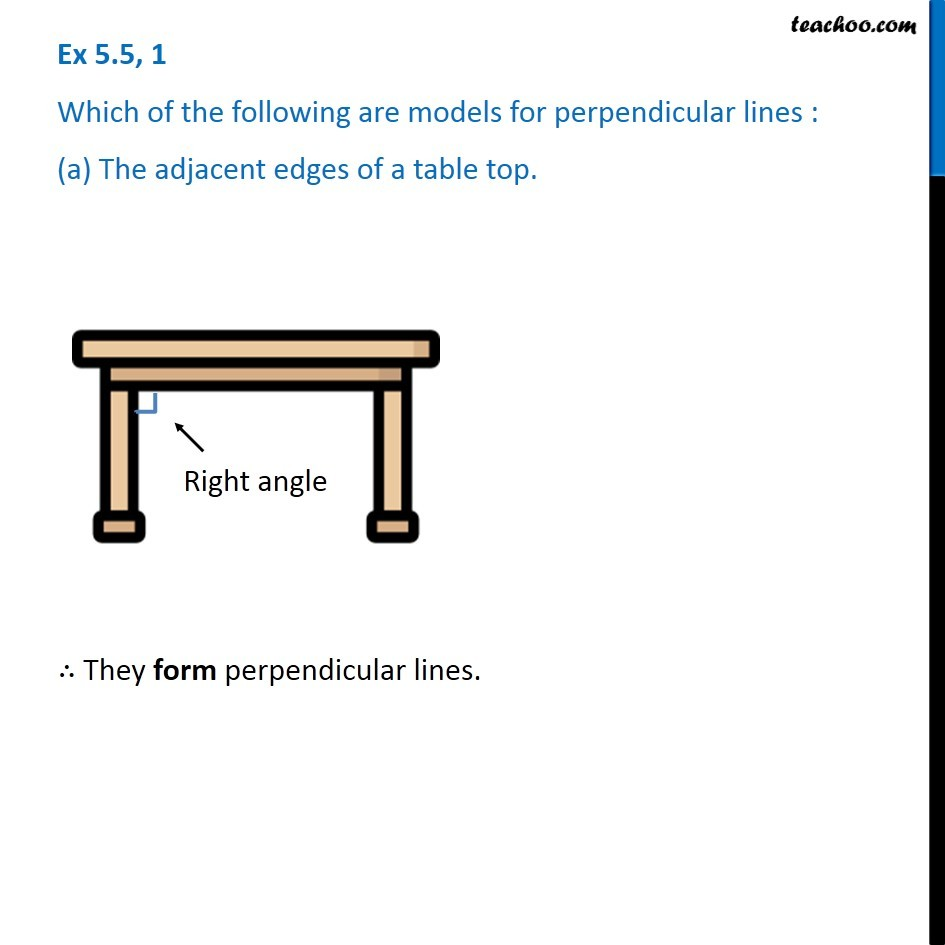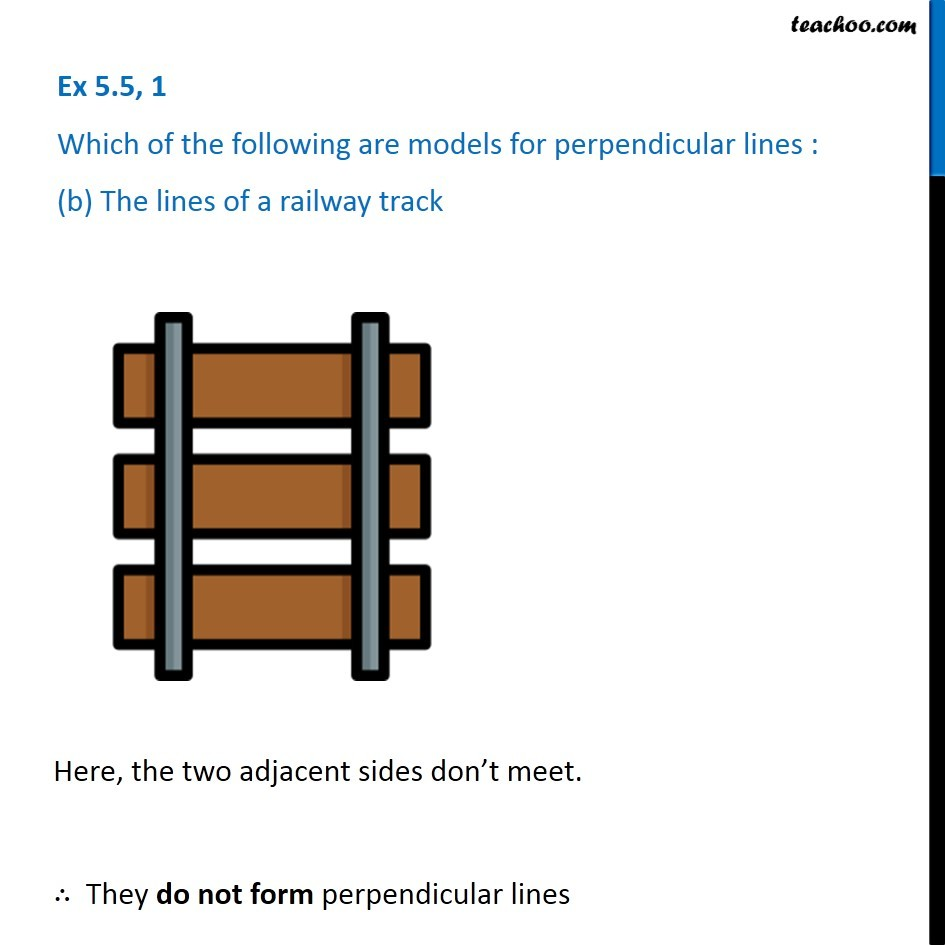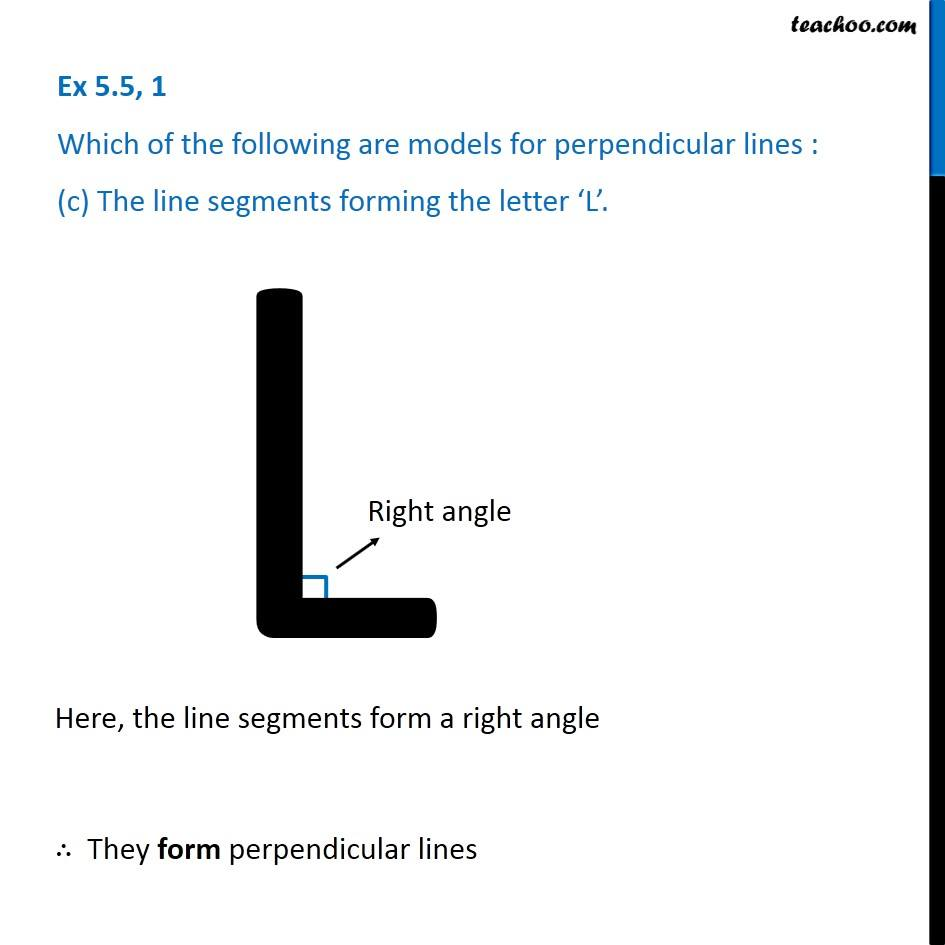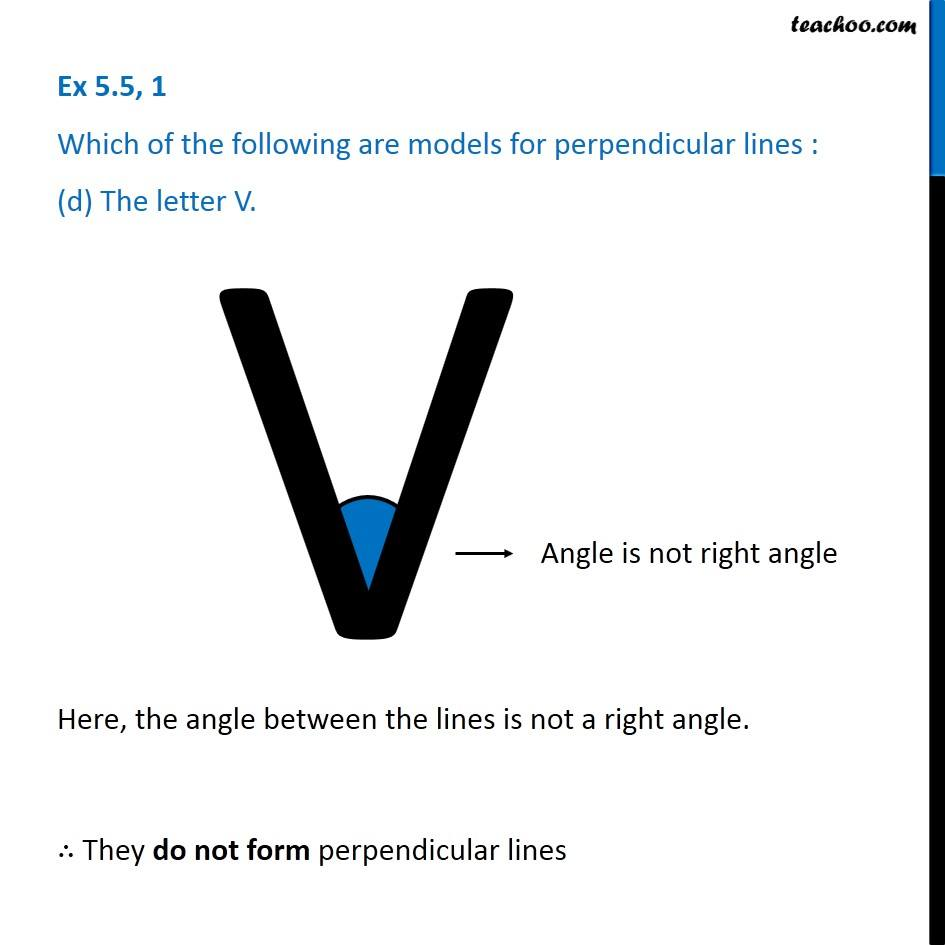Ex 5.5

Chapter 5 Class 6 Understanding Elementary Shapes
Serial order wise### Transcript

Ex 5.5, 1 Which of the following are models for perpendicular lines : (a) The adjacent edges of a table top. ∴ They form perpendicular lines. Ex 5.5, 1 Which of the following are models for perpendicular lines : (b) The lines of a railway track Here, the two adjacent sides don’t meet. ∴ They do not form perpendicular lines Ex 5.5, 1 Which of the following are models for perpendicular lines : (c) The line segments forming the letter ‘L’. Here, the line segments form a right angle ∴ They form perpendicular lines Ex 5.5, 1 Which of the following are models for perpendicular lines : (d) The letter V. Here, the angle between the lines is not a right angle. ∴ They do not form perpendicular lines